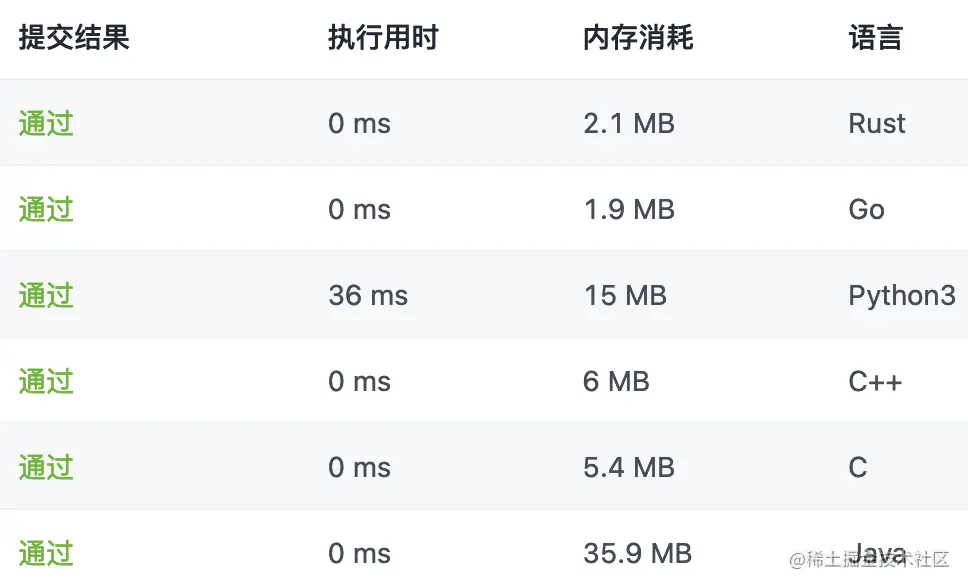# [algorithm learning] 1221 Split balanced string (Java / C / C + + / Python / go / trust)

2022-01-30 16:27:26

Thank you very much for reading this article ~ welcome 【 give the thumbs-up 】【 Collection 】【 Comment on 】~ It's not hard to give up , But persistence must be cool ~ I hope all of us can make a little progress every day ~ This paper is written by The white hat of the second leader https://juejin.cn/user/2771185768884824/posts Original blog ~

# 1221. Split balanced string ：

In a Balanced string in ,'L' and 'R' The number of characters is the same .

Give you a balanced string s, Please split it into as many balanced strings as possible .

Be careful ： Each split string must be a balanced string , And the balanced string obtained by segmentation is a continuous substring of the original balanced string .

Returns a balanced string that can be split The largest number .

# Examples 1

`````` Input ：
s = "RLRRLLRLRL"
Output ：
4
explain ：
s  Can be divided into  "RL"、"RRLL"、"RL"、"RL" , Each substring contains the same number of  'L'  and  'R' .
Copy code ``````

# Examples 2

`````` Input ：
s = "RLLLLRRRLR"
Output ：
3
explain ：
s  Can be divided into  "RL"、"LLLRRR"、"LR" , Each substring contains the same number of  'L'  and  'R' .
Copy code ``````

# Examples 3

`````` Input ：
s = "LLLLRRRR"
Output ：
1
explain ：
s  Just keep it as it is  "LLLLRRRR".
Copy code ``````

# Examples 4

`````` Input ：
s = "RLRRRLLRLL"
Output ：
2
explain ：
s  Can be divided into  "RL"、"RRRLLRLL" , Each substring contains the same number of  'L'  and  'R' .
Copy code ``````

# Tips

• 1 <= s.length <= 1000
• s[i] = 'L' or 'R'
• s It's a Balance character string

# analysis

• This algorithm problem if you say s If it is not a balanced string, it will be a little difficult .
• because s Itself is a balanced string , So you can use greed from left to right , The shorter it is, the more it can be divided , And the rest of the string is also a balanced string .
• We need to record the traversal process ‘L’ and ‘R’ The number of , If equal, it is a balanced string .
• Record separately ‘L’ and ‘R’ Quantity required 2 A variable , But we can record their difference , The difference is 0 It means that the quantity is equal , That is, the balanced string , In this way, only one variable is needed to record .

## java

``````class Solution {
public int balancedStringSplit(String s) {
int ans = 0;
//  Record the difference between left and right , Left subtraction , Right plus , by 0 The left and right numbers are the same
int v = 0;
int n = s.length();
for (int i = 0; i < n; ++i) {
if (s.charAt(i) == 'L') {
--v;
} else {
++v;
}
if (v == 0) {
++ans;
}
}
return ans;
}
}
Copy code ``````

## c

``````int balancedStringSplit(char * s){
int ans = 0;
//  Record the difference between left and right , Left subtraction , Right plus , by 0 The left and right numbers are the same
int v = 0;
while (*s) {
*s == 'L' ? --v : ++v;
if (v == 0) {
++ans;
}
++s;
}
return ans;
}
Copy code ``````

## c++

``````class Solution {
public:
int balancedStringSplit(string s) {
int ans = 0;
//  Record the difference between left and right , Left subtraction , Right plus , by 0 The left and right numbers are the same
int v = 0;
for (char c : s) {
c == 'L' ? --v : ++v;
if (v == 0) {
++ans;
}
}
return ans;
}
};
Copy code ``````

## python

``````class Solution:
def balancedStringSplit(self, s: str) -> int:
ans = 0
#  Record the difference between left and right , Left subtraction , Right plus , by 0 The left and right numbers are the same
v = 0
for c in s:
if c == 'L':
v -= 1
else:
v += 1
if v == 0:
ans += 1
return ans
Copy code ``````

## go

``````func balancedStringSplit(s string) int {
ans := 0
//  Record the difference between left and right , Left subtraction , Right plus , by 0 The left and right numbers are the same
v := 0
for _, c := range s {
if c == 'L' {
v--
} else {
v++
}
if v == 0 {
ans++
}
}
return ans
}
Copy code ``````

## rust

``````impl Solution {
pub fn balanced_string_split(s: String) -> i32 {
s.chars().scan(0, |v, c| {
match c {
'L' => *v -= 1,
_ => *v += 1
};
Some(*v)
}).filter(|v| { *v == 0 }).count() as i32
}
}
Copy code ``````# Original title transmission gate ：https://leetcode-cn.com/problems/split-a-string-in-balanced-strings/

Welcome to discuss in the comment area , Nuggets officials will be in Digging force Star Program After the event , Draw... In the comment area 100 Around the Nuggets , For details of the lucky draw, see the activity article